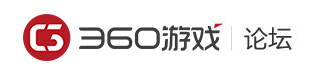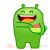### > 剑魂之刃 > 那么现在问题来了！来吧 不吐不快

7江湖少侠

7江湖少侠
。。。。。10一派堂主

6名动一方

6名动一方

7江湖少侠

7江湖少侠

VIP2=13%
VIP3=12%
VIP4=11%
VIP5=10%
VIP6=9%
VIP7=8%
VIP8=7%
VIP9=6%
VIP10=5%
VIP11=4%
VIP12=3%
VIP13=2%
VIP14=1%
........

6名动一方

7江湖少侠

VIP2=13%
VIP3=12%
VIP4=11%
VIP5=10%
VIP6=9%
VIP7=8%
VIP8=7%
VIP9=6%
VIP10=5%
VIP11=4%
VIP12=3%
VIP13=2%
VIP14=1%
........

9江湖豪侠

 您需要登录后才可以回帖 登录 | 立即注册 回帖后跳转到最后一页